# Room square

A Room square of side,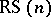, is an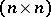-arraydefined on an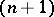-setwith the following properties:

1) each cell ofis either empty or contains an unordered pair of distinct elements from;

2) each element ofoccurs precisely once in each row and column of;

3) every unordered pair of elements fromis in precisely one cell of. A necessary condition for a Room square of sideto exist is thatbe odd. An example of a Room square of sideis listed below.

<tbody> </tbody>0 1 5 4 6 2 3 3 41 2 6 5 0 6 1 4 52 3 0 0 2 5 63 4 1 5 2 1 3 6 04 6 3 2 4 0 15 0 4 3 5 1 26

Room squares were named after T.G. Room, who published a paper in 1955 in which he constructed a Room square of sideand proved that Room squares of sideanddo not exist. However, Room squares were actually constructed much earlier. In 1850, T.M. Kirkman used a Room square of sideto solve the Kirkman schoolgirl problem for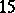girls, and the first infinite classes of Room squares were constructed by R.R. Anstice in 1852– 1853 [a1]. Several small Room squares were also constructed by E.C. Howell for use as schedules for duplicate bridge tournaments at the end of the nineteenth century. The existence of Room squares was finally completed in the early 1970s by R.C. Mullin and W.D. Wallis [a4]: A Room square of sideexists if and only ifis odd and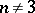or. The proof uses a number of direct and recursive constructions. An extensive literature on Room squares is available, see [a3].

One application of Room squares is in the construction of round robin tournaments. A Room square of sidecan be used to construct a round robin tournament withteams which has the following properties: every team plays every other team exactly once during the tournament; every team plays in exactly one game in each round; and every team plays at every location exactly once.

A Room square of sideis standardized with respect to the elementif cell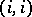contains the pair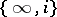. A standardized Room square of sideis skew if for every pair of cellsand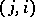(with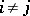) exactly one is filled. The existence of skew Room squares has been established [a5]: There exists a skew Room square of sideif and only ifis odd andor. Skew Room squares have been quite useful in constructions for several other types of combinatorial designs, see [a3].

Room squares with additional properties have also been studied; these include Room squares with sub-Room squares (incomplete Room squares), maximum empty subarray Room squares, perfect Room squares, and balanced Room squares (complete balanced Howell rotations), see [a3] for references. For generalizations of Room squares to larger block size and higher dimension, see Design with mutually orthogonal resolutions.

Room squares are equivalent to several other combinatorial configurations, [a2]. In particular, the existence of the following designs are equivalent:

a) a Room square of side;

b) a Room frame of type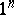;

c) two pairwise orthogonal symmetric Latin squares of order n (see Latin square);

d) a Howell design,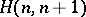;

e) two pairwise orthogonal one-factorizations of(cf. also One-factorization);

f) two orthogonal resolutions of an-BIBD (see Design with mutually orthogonal resolutions).

In some of the earlier literature, Room squares are also called Room designs.